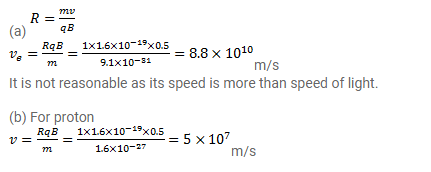# (a) An electron moves along a circle of radius 1m

Question:

(a) An electron moves along a circle of radius $1 \mathrm{~m}$ in a perpendicular magnetic field of strength $0.50 \mathrm{~T}$. What would be its speed? Is it reasonable?

(b) If a proton moves along a circle of same radius in the same magnetic field, what would be its speed?

Solution: